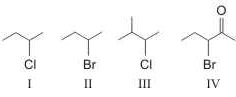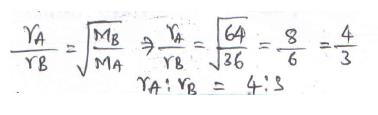Courses

# SRMJEEE Chemistry Mock Test - 10

## 35 Questions MCQ Test SRMJEEE Subject Wise & Full Length Mock Tests | SRMJEEE Chemistry Mock Test - 10

Description
This mock test of SRMJEEE Chemistry Mock Test - 10 for JEE helps you for every JEE entrance exam. This contains 35 Multiple Choice Questions for JEE SRMJEEE Chemistry Mock Test - 10 (mcq) to study with solutions a complete question bank. The solved questions answers in this SRMJEEE Chemistry Mock Test - 10 quiz give you a good mix of easy questions and tough questions. JEE students definitely take this SRMJEEE Chemistry Mock Test - 10 exercise for a better result in the exam. You can find other SRMJEEE Chemistry Mock Test - 10 extra questions, long questions & short questions for JEE on EduRev as well by searching above.
QUESTION: 1

Solution:
QUESTION: 2

Solution:
QUESTION: 3

### Which of the following monosaccharides is a pentose?

Solution:
QUESTION: 4

AgCl is soluble in

Solution:
QUESTION: 5

On hydrolysis of chloroform with aqueous potassium hydroxide, the final product obtained is

Solution:
QUESTION: 6

The number of unpaired electrons in Ni⁺⁺ is

Solution:
QUESTION: 7

Mixture of sand and sulphur may best be separated by

Solution:
QUESTION: 8

Reaction of benzene with alkyl halide in the presence of anhydrous AlCl3 is called

Solution:
QUESTION: 9

The greater the s- character in an orbital the ... is its energy

Solution:

The greater the s- character in an orbital, the shorter is the bond and greater is its strength. Thus, bond formed by sp hybridisation , as in acetylene is stronger as compare to ethane and ethene , because it has maximum bond energy.

QUESTION: 10

A carboxylic acid is converted into its anhydride using

Solution:
QUESTION: 11

Inter molecular forces in solid hydrogen are

Solution:
QUESTION: 12

When Zn dust is added to sufficienly large volume of aqueous solution of copper sulphate 3.175 g of copper metal and 20 J of heat is evolved. ∆H of reaction permol of Cu is

Solution:
QUESTION: 13

Which of these does not influence the rate of reaction?

Solution:
QUESTION: 14

For reaction at equilibrium, Le Chatelier's principle predicts the effects of

Solution:
QUESTION: 15

Identify the reaction for which ΔH ≠ ΔE

Solution:
QUESTION: 16

2, 4, 6-Trinitrophenol is a/an

Solution:
QUESTION: 17

What is the order of stability for
I.1-butene; II.cis-2-butene; III.trans-2-butene

Solution:
QUESTION: 18

Let electronegativity, ionisation energy and electron affinity be represented as EN, IP and EA respectively. Which one of the following equation is correct according to Mulliken?

Solution:

Mulliken-Jaffe Electronegativity:
Electronegativity (EN) can be regarded as the average of the ionisation energy (IE) and the electron affinity (EA) of an atom.
EN = IP + EA/2

QUESTION: 19

Pressure of a gas is due to

Solution:
QUESTION: 20

Standard reduction potentials of Sn2+ | Sn and Zn2+ | Zn electrodes are -0.14 V and -0.74 V respectively. What is the Eº of the following cell in volts?

Zn | Zn2+ || Sn2+ | Sn

Solution:
QUESTION: 21

Which of the following will have fastest rate of reaction with Br₂/FeBr₃?

Solution:
QUESTION: 22

To suppress the dissociation of acetric acid , the compound to be added to it is

Solution:
QUESTION: 23

Which among the following will not show chain isomerism?

Solution:
QUESTION: 24

Target nucleus A is converted to product nucleus B by (p, n) reaction : A (p, n) B.

Solution:
QUESTION: 25

When benzenediazonium chloride is treated with water, the compound formed is

Solution:
QUESTION: 26

Which of the following is a strongest base?

Solution:
QUESTION: 27

Arrange these compounds in order of increasing SN2 reaction rate :Solution:
QUESTION: 28

Which of the following is a bio-degradable polymer?

Solution:
QUESTION: 29

For the separation of two immiscible liquids which method is used ?

Solution:
QUESTION: 30

A compound contains atoms of three elements : A,B and C. If the oxidation number of A is + 2, B is + 5 and that of C is -2, the possible formula of the compound is

Solution:
QUESTION: 31

The major binding force of diamond, silicon and quartz is

Solution:
QUESTION: 32

Volume of 0.1 M K₂Cr₂O₇ required to oxidise 35 ml of 0.5 M FeSO₄ solution is

Solution:
QUESTION: 33

The rates of diffusion of gases A and B of molecular weight 36 and 64 are in the ratio

Solution:QUESTION: 34

The formula of metal chloride is MCl3. It contains 20% of metal. The atomic weight of metal is approximately

Solution:
QUESTION: 35

Which of the following type of molecules from micelles?

Solution: